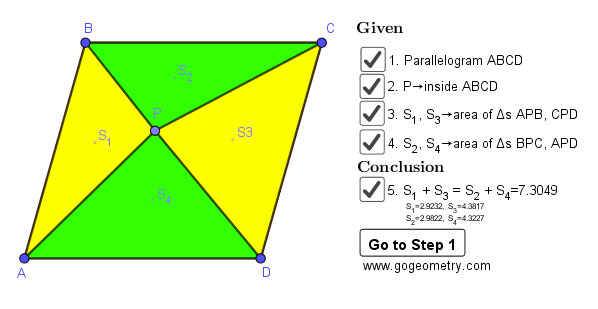# Dynamic Geometry Problem 1463: Parallelogram, Interior Point, Opposite Triangles with Equal Sum of Areas.

Let ABCD be a parallelogram with a point P inside ABCD (see the figure below). If S1 = Area APB, S3 = Area CPD, S2 = Area BPC, and S4 = Area APD, prove that S1 + S3 = S2 + S4.

## Static Diagram of Geometry Problem 1463## Poster of Geometry Problem 1463 using iPad Apps### Classroom Resource:Interactive step-by-step animation using GeoGebra

This step-by-step interactive illustration was created with GeoGebra.

• To explore (show / hide): click/tap a check box.
• To stop/play the animation: click/tap the icon in the lower left corner.
• To go to first step: click/tap the "Go to step 1" button.
• To manipulate the interactive figure: click/tap and drag the blue points or figures.

GeoGebra is free and multi-platform dynamic mathematics software for all levels of education that joins geometry, algebra, tables, graphing, statistics and calculus application, intended for teachers and students. Many parts of GeoGebra have been ported to HTML5.